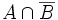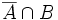# Varying normality

This is a survey article describing variations on the following: normal space
View other variational survey articles | View other survey articles about normal space

Normality is one of the most important properties of topological spaces; assuming that a topological space is normal can help us a long way. On the other hand, there are a umber of ways in which normality fails to be nice, and variants of normality have been cooked up to remedy this.

For a reasonably full list of variations, refer:

This article surveys some of the common variations of normality, trying to organize them into themes and streams. There are three basic ideas behind variation:

• Emulate the strengths
• Remedy the weaknesses
• Weaken or remove the strengths

Also refer the variational chart for variations stronger than normality.

File:Varynormality.jpg
Variations stronger than the property of normality

## What is separated

Normal spaces are spaces in which any two closed subsets can be separated by disjoint open subsets. One can vary this by controlling how many, and what kind of, subsets get separated.

### Weakening from closed subsets

Further information: Pseudonormal space, Regular space

A pseudonormal space is a T1 space in which any two closed subsets, of which one is at most countable in cardinality, can be separated by disjoint open sets. A regular space is a T1 space in which any two closed subsets, of which one is a point, can be separated by disjoint open sets.

Weakening from normality to regularity has both advantages and disadvantages. Regularity is hereditary; any subspace of a regular space is regular. Also, any product of regular spaces is regular. On the other hand, one cannot use separation by open sets to deduce separation by a continuous function for regular spaces (or for pseudonormal spaces, for that matter).

### Strengthening from closed subsets

Further information: hereditarily normal space

Instead of requiring any two closed subsets to be separated by disjoint open sets, we can strengthen the requirement: given any two subsets$A$ and$B$ such that$A \cap \overline{B}$ and$\overline{A} \cap B$ are both empty, there exist disjoint open sets$U, V$ containing$A$ and$B$ respectively.

This property is equivalent to being hereditarily normal, i.e. every subspace being normal. We shall encounter it a little later.

### Increasing the number of subsets

Further information: collectionwise normal space

A collectionwise normal space is a space in which not just two, but an arbitrary number of closed subsets can be separated by pairwise disjoint open sets, provided that they form a discrete collection; in other words, each of them is open in their union. It turns out that many of the spaces which we show to be normal, can actually be shown to be collectionwise normal. For instance, all metrizable spaces are collectionwise normal.

### Pre-determining the open sets in a compatible fashion

Further information: monotonically normal space

This is one of the least obvious, but most important variations of normality. A monotonically normal space is a T1 space where, to every pair of disjoint closed sets, we associate a way of separating them, such that this way of separating is monotone: if the closed set on one side gets bigger and that on the other side gets smaller, then the corresponding open sets also become bigger and smaller. It turns out that metrizable spaces are monotonically normal. The property of being monotonically normal is nice in a number of ways: it is hereditary, and it implies collectionwise normality.

Apart from metrizable spaces, elastic spaces and linearly orderable spaces are monotonically normal.

## Continuous functions and closed sets in terms of open sets

Further information: perfectly normal space

One way of viewing normality is that it shows that closed sets can be approximated by open sets. This is the essence of the idea of separating closed sets by continuous functions. A better question is: under what conditions can we guarantee that given two closed sets, there is a continuous function such that the inverse image of 0 is exactly one closed set, and the inverse image of 1 is exactly the other closed set? It turns out that this is equivalent to the property of being perfectly normal: the topological space is normal, and every closed set is a countable intersection of open subsets (called a G-delta subset).

The property of being perfectly normal inherits to subsets, hence perfectly normal spaces are hereditarily normal. Moreover, any metrizable space, and any CW-space, is perfectly normal.

## Remedying the defects of normality

### Lack of hereditariness

Normality is only weakly hereditary: any closed subset of a normal space is normal. There exist normal spaces with non-closed subsets, which are not normal. The simplest way to remedy this is to consider hereditarily normal spaces, which are topological spaces in which every subspace is normal. In practice, the following are two useful properties which imply being hereditarily normal:

Most of the usual cases where a space is hereditarily normal, it can be shown to be one or both of the above. For instance, metrizable spaces are both perfectly normal and monotonically normal. CW-spaces are perfectly normal (I'm not sure if they are monotonically normal). Linearly orderable spaces and ordered field-metrizable spaces are monotonically normal (there exist linearly orderable spaces that are not perfectly normal).

### Lack of closure under taking products

A product of normal spaces need not be normal. Moreover, even if we assume one of the spaces to be really nice, the other space could be bad enough for the product to not be normal. A notion which comes up in this context is binormal space: a normal space whose product with the unit interval is also normal.

Since a product of normal spaces need not be normal, we try for the next best thing: consider properties which imply normality, but which are closed under taking products. Note that we require both the spaces to satisfy the stronger property. Some examples of such properties are:

• Metrizable space: A finite product of metrizable spaces is metrizable, and any metrizable space is normal
• Compact Hausdorff space: An arbitrary product of compact Hausdorff spaces is compact Hausdorff.

There are also properties stronger than normality which are not closed under products, but whose products with compact Hausdorff spaces continue to satisfy the property. Examples are:

• Paracompact Hausdorff space: The product of a paracompact Hausdorff space with a compact Hausdorff space is paracompact Hausdorff, hence normal. In particular, paracompact Hausdorff spaces are binormal.
• Regular Lindelof space: The product of a regular Lindelof space with a compact Hausdorff space is regular Lindelof, hence normal.